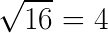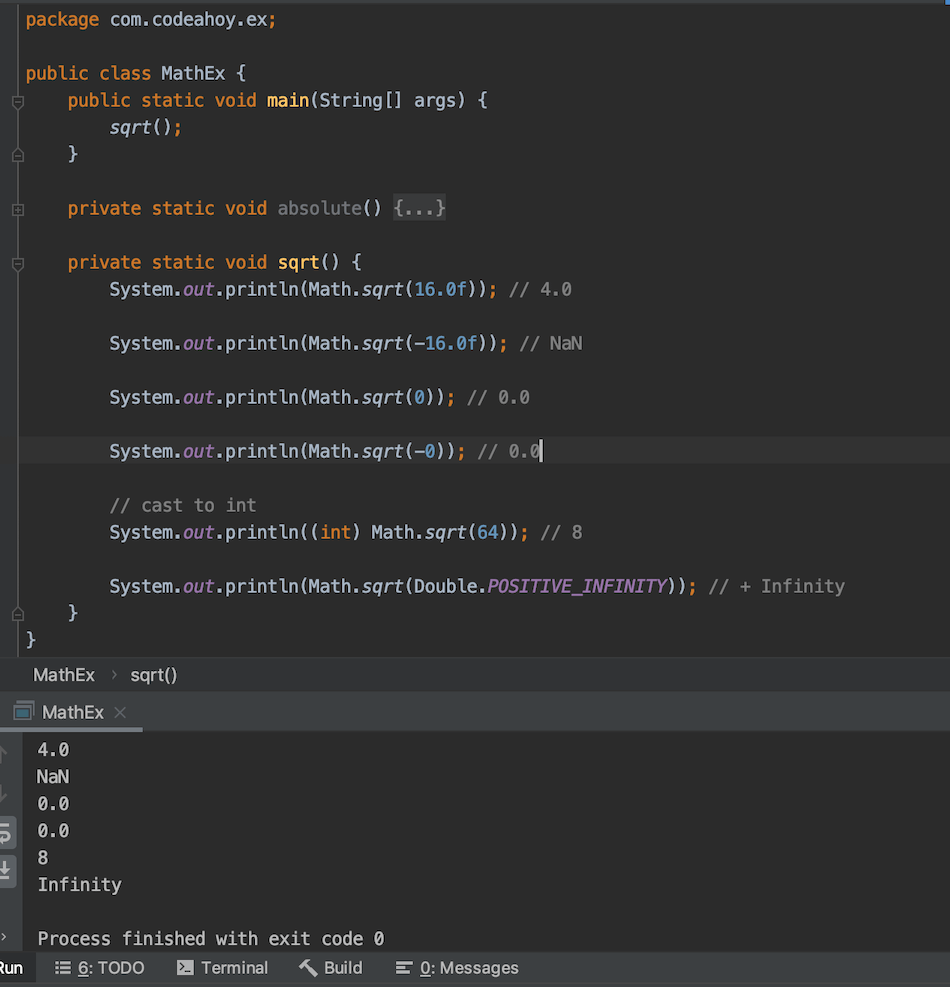# Java Math.sqrt() Method with Examples

Oct 12, 2019 · 2 mins readThe java.lang.Math class that comes bundled with Java contains various methods for performing basic numeric operations such as the elementary exponential, logarithm, square root, and trigonometric functions.

Math.sqrt(…) method is part of the Math class. We can use it to find the square root of a number. A square root of a number x is a number y such that y^2 = x. For example, 4 and −4 are square roots of 16 because 4^2 = (−4)^2 = 16Let’s look at its method signature:

``````public static double sqrt(double a)
``````

As we can see, it takes an argument of type `double` and returns it’s rounded square root, also as a `double`. Note just like all other methods on the Math class, Math.sqrt(…) is a `static` method so you can call it directly on the Math class.

``````int result = Math.sqrt(16.0); // result = 4.0
``````

If the argument you pass to the Math.sqrt(…) method is negative (less than 0,) it will return `NaN` (Not a number.)

``````int result = Math.sqrt(-16.0); // result = NaN
``````

## Code Example

``````public class MathEx {
public static void main(String[] args) {
sqrt();
}

private static void sqrt() {
System.out.println(Math.sqrt(16.0f)); // 4.0

System.out.println(Math.sqrt(-16.0f)); // NaN

System.out.println(Math.sqrt(0)); // 0.0

System.out.println(Math.sqrt(-0)); // 0.0

// cast to int
System.out.println((int) Math.sqrt(64)); // 8

System.out.println(Math.sqrt(Double.POSITIVE_INFINITY)); // +Inf
}
}
``````

Output

``````4.0
NaN
0.0
0.0
8
Infinity
``````

Here’s a screenshoot of the code above if you’re on mobile and having trouble viewing.### Special cases

• If the argument is NaN or less than zero, then the result is NaN.
• If the argument is positive infinity, then the result is positive infinity.
• If the argument is positive zero or negative zero, then the result is the same as the argument.

If you need more detailed information, please see Javadocs. It’s worth noting that the `Math` class in Java contains several other useful methods for arithmetic, logarithms and trignometric operations.

Here’s a short youtube video that walks through several methods of the Math class.

#### You May Also Enjoy

If you like this post, please share using the buttons above. It will help CodeAhoy grow and add new content. Thank you!